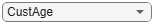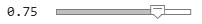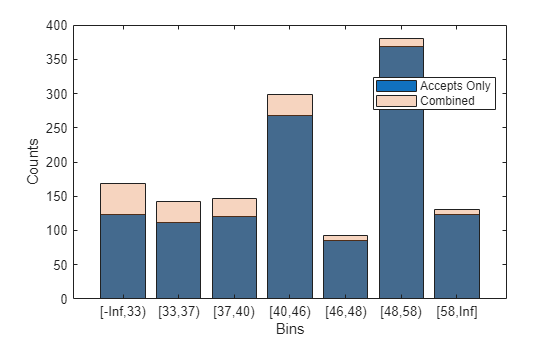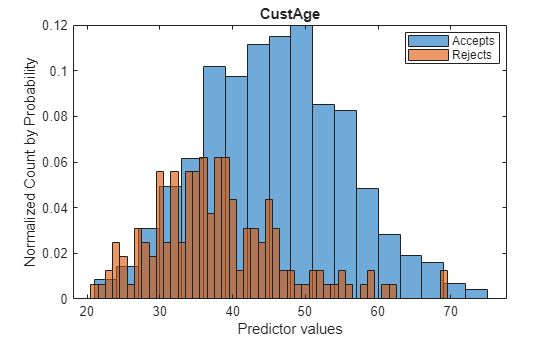# Use Reject Inference Techniques with Credit Scorecards

This example demonstrates the hard-cutoff and fuzzy augmentation approaches to reject inference.

Reject inference is a method for improving the quality of a credit scorecard by incorporating data from rejected loan applications. Bias can result if a credit scorecard model is built only on accepts and does not account for applications rejected because of past denials for credit or unknown nondefault status. By using the reject inference method, you can infer the performance of rejects and include them in your credit scorecard model to remedy this bias.

To develop a credit scorecard, you must identify each borrower as either "good" or "bad". For rejected applications, information to identify borrowers as "good" or "bad" is not available. You cannot tell for sure to which group a borrower would have belonged had they been granted a loan. The reject inference method allows you to infer whether a borrower would likely be "good" or "bad" enabling you to incorporate the rejected application data into the data set that you use to build a credit scorecard.

As the diagram shows, reject inference requires that you determine the threshold (cutoff point) below which rejects are considered as "bad." This example demonstrates the hard-cutoff and the fuzzy augmentation approaches to calculate this threshold.The following diagram shows the typical process for building a scorecard model. The red box represents the reject inference process, where the performance of the previously rejected applications is estimated and then used to re-train the credit scorecard model.The workflow for the reject inference process is:

1. Build a logistic regression model based on the accepts.

2. Infer the class of rejects using one of the reject inference techniques.

3. Combine the accepts and rejects into a single data set.

4. Create a new scorecard, bin the expanded data set, and build a new logistic model.

5. Validate the final model.

There are two types of reject inference:

• Simple assignment does not use a reject inference process and either ignores rejects or assigns all rejects to the "bad" class.

• Augmentation uses a reject inference process to handle rejects based on a scoring model by combining the original data set with the rejects data.

This example focuses on augmentation techniques. The most popular techniques for augmentation are:

• Simple augmentation — Using a cutoff value, this method assigns rejects with scores below and above the value to the "bad" or "good" class, respectively. The cutoff value must reflect that the rate of bads in the rejects is higher than in the accepted population. After the class ("good" or "bad") is assigned to the rejects, the entire population of accepts and rejects are fitted in the credit scorecard model and then scored. This approach is also called the hard-cutoff technique.

• Fuzzy augmentation — This method scores the rejects by using a credit scorecard model based on the accepts. These rejects are duplicated into two observations, where each is assigned a probability of being "good" or "bad," and then aggregated to the accepts. A new credit scorecard model is then estimated on the new data set.

In this example, the following workflows are presented:

Both of these approaches use the binning rules preserved from the original scorecard and apply them to the new scorecard that is based on the combined data set.

Note: The data sets in this example are technically through-the-door (TTD) observations. That is, accepts and rejects are lumped together and differentiated based on their accept or reject decision. A rejects data set is then created from the TTD observations.

### Hard-Cutoff Technique Workflow

The hard-cutoff technique uses a predefined cutoff value and assigns rejects below the cutoff as "bad" and above the cutoff as "good." The cutoff value must reflect that the rate of "bads" in the rejects is higher than in the accepts. After each reject is assigned a class ("good" or "bad"), the entire population of accepts and rejects is fitted in a credit scorecard model, and then that model is scored and validated. This approach is also called the simple augmentation technique. The main challenge in this approach is choosing the cutoff value.

First, visualize the data for accepts and rejects for a selected predictor.

```% Load the data load CreditCardData.mat load RejectsCreditCardData.mat Predictor ="CustAge"; figure; h1 = histogram(data.(Predictor)); hold on h2 = histogram(Rejects.(Predictor)); h1.Normalization = 'probability'; h2.Normalization = 'probability'; title(Predictor) xlabel('Predictor Values') ylabel('Normalized Count by Probability') hold off legend({'Accepts','Rejects'},'Location','best');```#### Create a `creditscorecard` Object for the Accepts and Score the Data

Use `creditscorecard` to create a `creditscorecard` object that you can use to bin, fit, and then score the accepts.

```scHC = creditscorecard(data,'IDVar','CustID'); scHC = autobinning(scHC); scHC = fitmodel(scHC);```
```1. Adding CustIncome, Deviance = 1490.8527, Chi2Stat = 32.588614, PValue = 1.1387992e-08 2. Adding TmWBank, Deviance = 1467.1415, Chi2Stat = 23.711203, PValue = 1.1192909e-06 3. Adding AMBalance, Deviance = 1455.5715, Chi2Stat = 11.569967, PValue = 0.00067025601 4. Adding EmpStatus, Deviance = 1447.3451, Chi2Stat = 8.2264038, PValue = 0.0041285257 5. Adding CustAge, Deviance = 1441.994, Chi2Stat = 5.3511754, PValue = 0.020708306 6. Adding ResStatus, Deviance = 1437.8756, Chi2Stat = 4.118404, PValue = 0.042419078 7. Adding OtherCC, Deviance = 1433.707, Chi2Stat = 4.1686018, PValue = 0.041179769 Generalized linear regression model: logit(status) ~ 1 + CustAge + ResStatus + EmpStatus + CustIncome + TmWBank + OtherCC + AMBalance Distribution = Binomial Estimated Coefficients: Estimate SE tStat pValue ________ ________ ______ __________ (Intercept) 0.70239 0.064001 10.975 5.0538e-28 CustAge 0.60833 0.24932 2.44 0.014687 ResStatus 1.377 0.65272 2.1097 0.034888 EmpStatus 0.88565 0.293 3.0227 0.0025055 CustIncome 0.70164 0.21844 3.2121 0.0013179 TmWBank 1.1074 0.23271 4.7589 1.9464e-06 OtherCC 1.0883 0.52912 2.0569 0.039696 AMBalance 1.045 0.32214 3.2439 0.0011792 1200 observations, 1192 error degrees of freedom Dispersion: 1 Chi^2-statistic vs. constant model: 89.7, p-value = 1.4e-16 ```
```ScoreRange = [300 850]; scHC = formatpoints(scHC,'WorstAndBestScores',ScoreRange); ScoresAccepts = score(scHC);```

#### Choose a Bad Rate and Score the Rejects

A reject is "good" or "bad" based on the specified bad rate (BR) value. In general, the credit scoring industry assumes that rejects have a BR of 75%. This is a subjective evaluation that is usually based on an unknown value. In this example, you can adjust the value of `BR`.

The `CreditCardData.mat` input data has `'status'` as response. Assume that `GoodLabel` (which means a nondefault) is the class that has a higher count in the response. In this example, `GoodLabel` is `0`, which means that default only happens when the response is equal to `1`.

```% Define the BR BR =0.75; % Sort rejects by ascending CustID order N = height(Rejects); Rejects = sortrows(Rejects); ScoresRejects = score(scHC,Rejects); % Find the lowest quantile based on the BR and set the corresponding observations to bad BadLabel = setdiff(unique(scHC.Data.(scHC.ResponseVar)),scHC.GoodLabel); ScoreThres = quantile(ScoresRejects,BR); ResponseRejects = zeros(N,1); ResponseRejects(ScoresRejects < ScoreThres) = BadLabel; ResponseRejects(ScoresRejects >= ScoreThres) = scHC.GoodLabel; % Create the rejects table RejectsTable = [Rejects table(ResponseRejects,'VariableNames',{scHC.ResponseVar})];```

#### Combine Accepts and Rejects Into a New Data Set, Score, and Validate

To draw a more accurate comparison between the accepts and the combined data set, use the same binning rules from the initial accepts credit scorecard and copy them to the `creditscorecard` object built on the combined dataset. This ensures that the binning assignment does not affect the later comparison of the two credit scorecard models. Also, you can visualize how the rejects are spread out in the data range of each predictor.

```% Create the final combined scorecard CombinedData = [data(:,2:end);RejectsTable(:,2:end)]; scNewHC = creditscorecard(CombinedData,'GoodLabel',0); % Bin using the same binning rules as the base scorecard Predictors = scHC.PredictorVars; Edges = struct(); for i = 1 : length(Predictors) Pred = Predictors{i}; [bi,cp] = bininfo(scHC,Pred); if ismember(Pred,scHC.NumericPredictors) scNewHC = modifybins(scNewHC,Pred,'CutPoints',cp); else scNewHC = modifybins(scNewHC,Pred,'CatGrouping',cp); end Edges.(Pred) = bi.Bin(1:end-1); end % Visualize the rejects distribution in each bin bd1 = bindata(scHC,data); bd2 = bindata(scHC,CombinedData); Predictor ="CustAge"; figure; bar(categorical(Edges.(Predictor)),histcounts(bd1.(Predictor))) hold on bar(categorical(Edges.(Predictor)),histcounts(bd2.(Predictor)),'FaceAlpha',0.25) hold off xlabel('Bins') ylabel('Counts') legend({'Accepts Only','Combined'},'Location','best')```Compare the initial `creditscorecard` object (`scHC`) to the new `creditscorecard` object (`scNewHC`) for the distribution of "goods" and "bads" for the selected predictor.

`plotbins(scHC,Predictor);``plotbins(scNewHC,Predictor);`Fit a logistic regression model for the `creditscorecard` object `scNewHC` and then score `scNewHC`.

`scNewHC = fitmodel(scNewHC);`
```1. Adding CustIncome, Deviance = 1693.9882, Chi2Stat = 114.39516, PValue = 1.0676416e-26 2. Adding TmWBank, Deviance = 1650.6615, Chi2Stat = 43.326628, PValue = 4.6323638e-11 3. Adding AMBalance, Deviance = 1623.0668, Chi2Stat = 27.594773, PValue = 1.4958244e-07 4. Adding EmpStatus, Deviance = 1603.603, Chi2Stat = 19.463733, PValue = 1.0252802e-05 5. Adding CustAge, Deviance = 1592.3467, Chi2Stat = 11.256272, PValue = 0.00079354409 6. Adding ResStatus, Deviance = 1582.0086, Chi2Stat = 10.338134, PValue = 0.0013030966 7. Adding OtherCC, Deviance = 1572.1, Chi2Stat = 9.9086387, PValue = 0.0016450476 Generalized linear regression model: logit(status) ~ 1 + CustAge + ResStatus + EmpStatus + CustIncome + TmWBank + OtherCC + AMBalance Distribution = Binomial Estimated Coefficients: Estimate SE tStat pValue ________ ________ ______ __________ (Intercept) 0.48115 0.061301 7.849 4.1925e-15 CustAge 0.50857 0.14449 3.5197 0.00043207 ResStatus 1.151 0.34773 3.3101 0.00093262 EmpStatus 0.78527 0.17826 4.4051 1.0572e-05 CustIncome 0.68743 0.12372 5.5563 2.7555e-08 TmWBank 1.0001 0.16731 5.9779 2.2607e-09 OtherCC 0.97659 0.30956 3.1548 0.0016062 AMBalance 0.91563 0.19073 4.8006 1.5819e-06 1361 observations, 1353 error degrees of freedom Dispersion: 1 Chi^2-statistic vs. constant model: 236, p-value = 2.29e-47 ```
```scNewHC = formatpoints(scNewHC,'WorstAndBestScores',ScoreRange); Scores = score(scNewHC); % Visualize the score distribution histogram(ScoresAccepts)```
```Warning: Error updating Legend. Property BaselineB_IS not found in class matlab.graphics.axis.PlotTarget, or is not present in all elements of the array of class matlab.graphics.axis.PlotTarget. ```
```Warning: Error updating Legend. Property BaselineB_IS not found in class matlab.graphics.axis.PlotTarget, or is not present in all elements of the array of class matlab.graphics.axis.PlotTarget. ```
```hold on histogram(Scores,'FaceAlpha',0.25) hold off ylabel('Counts') xlabel('Scores') title(sprintf('Score Distribution for a BR = %.2f',BR)) legend({'Accepts Only','Combined'},'Location','best')```#### Validate the Model on the Combined Data Set

Before validation, you must adjust the data set. To adjust the data set, you can either:

• Validate the accepts for both scorecards

• Validate the combined data set for both scorecards

```% Get statistics for the accepts StatsA1 = validatemodel(scHC);```
```Warning: Error updating Legend. Property BaselineB_IS not found in class matlab.graphics.axis.PlotTarget, or is not present in all elements of the array of class matlab.graphics.axis.PlotTarget. ```
```Warning: Error updating Legend. Property BaselineB_IS not found in class matlab.graphics.axis.PlotTarget, or is not present in all elements of the array of class matlab.graphics.axis.PlotTarget. ```
```Warning: Error updating Legend. Property BaselineB_IS not found in class matlab.graphics.axis.PlotTarget, or is not present in all elements of the array of class matlab.graphics.axis.PlotTarget. ```
```StatsA2 = validatemodel(scNewHC,data); % Get the statistics for the combined data set StatsC1 = validatemodel(scHC,CombinedData); StatsC2 = validatemodel(scNewHC); s1 = table(StatsA1.Value,StatsA2.Value,'VariableNames',{'BaseScorecard','CombinedScorecard'}); s2 = table(StatsC1.Value,StatsC2.Value,'VariableNames',{'BaseScorecard','CombinedScorecard'}); Stats = table(StatsA1.Measure,s1,s2,'VariableNames',{'Measure','Accepts','Combined'}); disp(Stats)```
``` Measure Accepts Combined BaseScorecard CombinedScorecard BaseScorecard CombinedScorecard ________________________ __________________________________ __________________________________ {'Accuracy Ratio' } 0.32258 0.31695 0.47022 0.46565 {'Area under ROC curve'} 0.66129 0.65848 0.73511 0.73282 {'KS statistic' } 0.2246 0.22946 0.34528 0.33681 {'KS score' } 550.72 576.57 512.44 542.85 ```

### Fuzzy Augmentation Technique Workflow

The Fuzzy augmentation technique starts by building a scorecard using the accepts only and then this scorecard model is used to score the rejects. Unlike the hard-cutoff technique, the fuzzy augmentation approach does not assign "good" or "bad" classes. Rather, each reject is duplicated into two observations and assigned a weighted "good" or "bad" value, based on a probability of being "good" or "bad." The weighted rejects are then added to the accepts data set and the combined data set is used to create a scorecard that is then fit and validated.

First, visualize the data for accepts and rejects for a selected predictor.

```% Load the data load CreditCardData.mat load RejectsCreditCardData.mat Predictor ="CustAge"; figure; h1 = histogram(data.(Predictor)); hold on h2 = histogram(Rejects.(Predictor)); h1.Normalization = 'probability'; h2.Normalization = 'probability'; title(Predictor) xlabel('Predictor values') ylabel('Normalized Count by Probability') hold off legend({'Accepts','Rejects'},'Location','best');```#### Create a `creditscorecard` Object for the Accepts and Score the Data

Use `creditscorecard` to create a `creditscorecard` object for the accepts, which you can bin, fit, and then score.

```scFA = creditscorecard(data,'IDVar','CustID'); scFA = autobinning(scFA); scFA = fitmodel(scFA);```
```1. Adding CustIncome, Deviance = 1490.8527, Chi2Stat = 32.588614, PValue = 1.1387992e-08 2. Adding TmWBank, Deviance = 1467.1415, Chi2Stat = 23.711203, PValue = 1.1192909e-06 3. Adding AMBalance, Deviance = 1455.5715, Chi2Stat = 11.569967, PValue = 0.00067025601 4. Adding EmpStatus, Deviance = 1447.3451, Chi2Stat = 8.2264038, PValue = 0.0041285257 5. Adding CustAge, Deviance = 1441.994, Chi2Stat = 5.3511754, PValue = 0.020708306 6. Adding ResStatus, Deviance = 1437.8756, Chi2Stat = 4.118404, PValue = 0.042419078 7. Adding OtherCC, Deviance = 1433.707, Chi2Stat = 4.1686018, PValue = 0.041179769 Generalized linear regression model: logit(status) ~ 1 + CustAge + ResStatus + EmpStatus + CustIncome + TmWBank + OtherCC + AMBalance Distribution = Binomial Estimated Coefficients: Estimate SE tStat pValue ________ ________ ______ __________ (Intercept) 0.70239 0.064001 10.975 5.0538e-28 CustAge 0.60833 0.24932 2.44 0.014687 ResStatus 1.377 0.65272 2.1097 0.034888 EmpStatus 0.88565 0.293 3.0227 0.0025055 CustIncome 0.70164 0.21844 3.2121 0.0013179 TmWBank 1.1074 0.23271 4.7589 1.9464e-06 OtherCC 1.0883 0.52912 2.0569 0.039696 AMBalance 1.045 0.32214 3.2439 0.0011792 1200 observations, 1192 error degrees of freedom Dispersion: 1 Chi^2-statistic vs. constant model: 89.7, p-value = 1.4e-16 ```
```ScoreRange = [300 850]; scFA = formatpoints(scFA,'WorstAndBestScores',ScoreRange); ScoresAccepts = score(scFA);```

#### Score the Rejects and Create the Combined Data Set

```% Load the rejects dataset and score the observations load RejectsCreditCardData.mat ScoresRejects = score(scFA,Rejects); % Compute the probabilities of default and use as weights pdRejects = probdefault(scFA,Rejects); % Assign bad status to pd (probability of default) and good status to 1-pd weights BadLabel = setdiff(unique(scFA.Data.(scFA.ResponseVar)),scFA.GoodLabel); Weights = zeros(2*length(pdRejects),1); Response = zeros(2*length(pdRejects),1); Weights(1:2:end) = pdRejects; Response(1:2:end) = BadLabel; Weights(2:2:end) = 1-pdRejects; Response(2:2:end) = scFA.GoodLabel; % Rearrange the response so that each two rows correspond to the same % observation from rejects RejectsTable = repelem(Rejects(:,2:end),2,1); RejectsTable = addvars(RejectsTable,Weights,Response,'NewVariableNames',... {'Weights',scFA.ResponseVar}); % Combine accepts and rejects AcceptsData = addvars(data,ones(height(data),1),'Before',scFA.ResponseVar,... 'NewVariableNames','Weights'); CombinedData = [AcceptsData(:,2:end);RejectsTable];```

#### Combine Accepts and Rejects into a New Data Set, Score, and Validate

To draw a more accurate comparison between the accepts and the combined data set, use the same binning rules from the initial accepts credit scorecard and copy them to the `creditscorecard` object built on the combined dataset. This ensures that the binning assignments does not affect the later comparison of the two credit scorecard models. Also, you can visualize how the rejects are spread out in the data range of each predictor.

```scNewFA = creditscorecard(CombinedData,'GoodLabel',0,'WeightsVar','Weights'); % Bin using the same binning rules as the base scorecard Predictors = scFA.PredictorVars; Edges = struct(); for i = 1 : length(Predictors) Pred = Predictors{i}; [bi,cp] = bininfo(scFA,Pred); if ismember(Pred,scFA.NumericPredictors) scNewFA = modifybins(scNewFA,Pred,'CutPoints',cp); else scNewFA = modifybins(scNewFA,Pred,'CatGrouping',cp); end Edges.(Pred) = bi.Bin(1:end-1); end % Visualize the rejects distribution in each bin bd1 = bindata(scFA,data); bd2 = bindata(scFA,CombinedData); Predictor ="CustAge"; figure; bar(categorical(Edges.(Predictor)),histcounts(bd1.(Predictor))) hold on bar(categorical(Edges.(Predictor)),histcounts(bd2.(Predictor)),'FaceAlpha',0.25) hold off xlabel('Bins') ylabel('Counts') legend({'Accepts Only','Combined'},'Location','best')```Compare the initial `creditscorecard` object (`scFA`) to the new `creditscorecard` object (`scNewFA`) for the distribution of "goods" and "bads" for the selected predictor.

`plotbins(scFA,Predictor);``plotbins(scNewFA,Predictor);`Fit a logistic regression model for the `creditscorecard` object `scNewFA` and then score `scNewFA`.

`scNewFA = fitmodel(scNewFA);`
```1. Adding CustIncome, Deviance = 1711.3102, Chi2Stat = 54.160619, PValue = 1.8475277e-13 2. Adding TmWBank, Deviance = 1682.5353, Chi2Stat = 28.774866, PValue = 8.1299351e-08 3. Adding AMBalance, Deviance = 1668.2956, Chi2Stat = 14.239727, PValue = 0.00016093686 4. Adding EmpStatus, Deviance = 1658.2944, Chi2Stat = 10.001236, PValue = 0.001564352 5. Adding CustAge, Deviance = 1652.3976, Chi2Stat = 5.8967925, PValue = 0.015168483 6. Adding OtherCC, Deviance = 1647.7632, Chi2Stat = 4.6344022, PValue = 0.031337059 7. Adding ResStatus, Deviance = 1642.8332, Chi2Stat = 4.9299914, PValue = 0.026394448 Generalized linear regression model: logit(status) ~ 1 + CustAge + ResStatus + EmpStatus + CustIncome + TmWBank + OtherCC + AMBalance Distribution = Binomial Estimated Coefficients: Estimate SE tStat pValue ________ ________ ______ __________ (Intercept) 0.60838 0.059654 10.198 2.0142e-24 CustAge 0.50755 0.20092 2.5262 0.011532 ResStatus 1.082 0.48919 2.2119 0.026971 EmpStatus 0.74776 0.23526 3.1784 0.0014809 CustIncome 0.6372 0.17519 3.6371 0.00027567 TmWBank 0.96561 0.19664 4.9106 9.0815e-07 OtherCC 0.90699 0.40476 2.2408 0.025039 AMBalance 0.87642 0.25404 3.4499 0.00056077 1522 observations, 1514 error degrees of freedom Dispersion: 1 Chi^2-statistic vs. constant model: 123, p-value = 2.16e-23 ```
```scNewFA = formatpoints(scNewFA,'WorstAndBestScores',ScoreRange); Scores = score(scNewFA); pd = probdefault(scNewFA); % Visualize the score distribution histogram(ScoresAccepts)```
```Warning: Error updating Legend. Property BaselineB_IS not found in class matlab.graphics.axis.PlotTarget, or is not present in all elements of the array of class matlab.graphics.axis.PlotTarget. ```
```Warning: Error updating Legend. Property BaselineB_IS not found in class matlab.graphics.axis.PlotTarget, or is not present in all elements of the array of class matlab.graphics.axis.PlotTarget. ```
```hold on histogram(Scores,'FaceAlpha',0.25) hold off ylabel('Counts') xlabel('Scores') title('Score Distribution Using Fuzzy Augmentation') legend({'Accepts Only','Combined'},'Location','best')```#### Validate the Model on the Combined Data Set

Before validation, you must adjust the data set. To adjust the data set, you can either:

• Validate the accepts for both scorecards

• Validate the combined data set for both scorecards

```% Get statistics for the accepts data.Weights = ones(height(data),1);```
```Warning: Error updating Legend. Property BaselineB_IS not found in class matlab.graphics.axis.PlotTarget, or is not present in all elements of the array of class matlab.graphics.axis.PlotTarget. ```
```Warning: Error updating Legend. Property BaselineB_IS not found in class matlab.graphics.axis.PlotTarget, or is not present in all elements of the array of class matlab.graphics.axis.PlotTarget. ```
```Warning: Error updating Legend. Property BaselineB_IS not found in class matlab.graphics.axis.PlotTarget, or is not present in all elements of the array of class matlab.graphics.axis.PlotTarget. ```
```Warning: Error updating Legend. Property BaselineB_IS not found in class matlab.graphics.axis.PlotTarget, or is not present in all elements of the array of class matlab.graphics.axis.PlotTarget. ```
```StatsA1 = validatemodel(scFA); StatsA2 = validatemodel(scNewFA,data); % Get the statistics for the combined data set StatsC1 = validatemodel(scFA,CombinedData); StatsC2 = validatemodel(scNewFA); s1 = table(StatsA1.Value,StatsA2.Value,'VariableNames',{'BaseScorecard','CombinedScorecard'}); s2 = table(StatsC1.Value,StatsC2.Value,'VariableNames',{'BaseScorecard','CombinedScorecard'}); Stats = table(StatsA1.Measure,s1,s2,'VariableNames',{'Measure','Accepts','Combined'}); disp(Stats)```
``` Measure Accepts Combined BaseScorecard CombinedScorecard BaseScorecard CombinedScorecard ________________________ __________________________________ __________________________________ {'Accuracy Ratio' } 0.32258 0.32088 0.29419 0.35143 {'Area under ROC curve'} 0.66129 0.66044 0.64709 0.67571 {'KS statistic' } 0.2246 0.22799 0.22596 0.25629 {'KS score' } 550.72 554.84 512.44 520.99 ```

### Summary

This example demonstrates how to use a reject inference process within the framework of the credit scorecard workflow. The Hard-Cutoff and the Fuzzy Augmentation techniques show how you can bin the data, fit a model, integrate the rejects with the accepts into a new credit scorecard model, and then validate the new credit scorecard model.

There is no clear-cut conclusion for which of these reject inference approaches is the best. This example is intended to illustrate how to use the features of `creditscorecard` to implement two different reject inference approaches.

### References

1. Baesesn, B., D. Rösch, and H. Scheule. Credit Risk Analytics: Measurement Techniques, Applications and Examples in SAS. Wiley and SAS Business Series, 2016.

2. Refaat, M. Credit Risk Scorecards: Development and Implementation Using SAS. lulu.com, 2011.